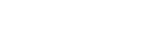# MATH112 - College Algebra

## General

MATH

112

00001820

Lecture

### Description

Functions and graphs; polynomial, radical, rational, exponential, logarithmic functions; equations, inequalities, systems of equations; applications. No more than 6 credits from MATH 112, 113, and 115 may be counted toward graduation. Prereq.: MATH 072 or high school advanced algebra with satisfactory math placement score.

### Notes

Go to www.stcloudstate.edu/mathplacement Placement req prior to Registration

3

3

No

Yes

04

No

## Learning Outcomes

### Outcome

Apply algebraic, graphical, numerical, and verbal skills to a variety of types of functions: linear, quadratic, polynomial, radical, rational, exponential, and logarithmic functions.

### Outcome

Analyze behaviors of functions considering domain and range, transformations of familiar functions, intercepts, symmetry, increasing and decreasing intervals, asymptotes, and relative minimum and maximum function values.

### Outcome

Find real and nonreal complex solutions to quadratic and polynomial equations using factoring, the Rational Zero Theorem, synthetic division, the quadratic formula, and other techniques.

### Outcome

Use logarithms to solve exponential equations, in the creation of exponential models, and to expand and condense logarithmic expressions.

### Outcome

Use the difference quotient or two points on the graph to determine the average rate of change of a function.

### Outcome

Solve systems of linear and nonlinear equations.

### Outcome

Communicate their knowledge of functions, equations, and inequalities, both orally (e.g. class discussions) and in writing (e.g. written assessments).

-

## Dependencies

No dependencies720 4th Avenue South
St. Cloud, MN 56301-4498
(320) 308-0121St. Cloud State University
A member of Minnesota State and committed to legal affirmative action, equal opportunity, access and diversity of its campus community (Full Statement).
© 2021 St. Cloud State University